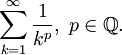# Final Thoughts on The Integral Test

Previous: Videos on the Integral Test

Next: The Alternating Series Test

The previous lesson on the divergence test gave us a way of determining whether some infinite series diverge. We saw that the divergence test had a limitation: it can tell us if certain infinite series diverges, but it cannot tell us if a given series converges. But there are other convergence tests. The integral test, for example, provides a test for any serieswhose terms an can be related to a continuous, positive, decreasing function. Essentially, we let, then evaluate the integraland:

• if the integral converges, the infinite series converges, and
• if the integral diverges, the infinite series diverges.

Although this test is limited to functions who are continuous, positive and decreasing, we saw that it led us to a useful convergence theorem for any infinite series of the formPrevious: Videos on the Integral Test

Next: The Alternating Series Test